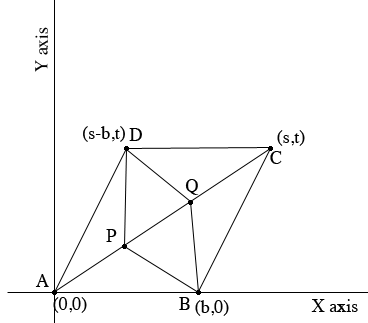SEARCH HOMEMath Central Quandaries & QueriesQuestion from Apollo: Prove analytically that if ABCD is a parallelogram in which points P and Q trisects the diagonal AC, then BPDQ is a parallelogram.Hi Apollo,

I drew a diagram in the Cartesian plane of what you described. I put $A$ at the origin, $B$ on the X-axis with coordinates (0,b), and $C$ at (s, t).Since $AB$ is parallel to $CD,$ and $BC$ is parallel to $AD,$ you can determine the coordinates of $C$ and $D.$

What are the coordinates of $P$ and $Q?$

To show that $BPDQ$ is a parallelogram find the slopes of its four sides.

PennyMath Central is supported by the University of Regina and The Pacific Institute for the Mathematical Sciences.